Home MATHEMATICS TOPIC 3: DECIMAL AND PERCENTAGE ~ MATHEMATICS FORM 1

# TOPIC 3: DECIMAL AND PERCENTAGE ~ MATHEMATICS FORM 1

185
1
SHAREDECIMAL AND PERCENTAGE ~ MATHEMATICS FORM 1

# DECIMAL AND PERCENTAGE ~ MATHEMATICS FORM 1

The Concept of Decimals
Explain the concept of decimals
A decimal- is defined as a number which consist of two parts separated by a point.The parts are whole number part and fractional part

#### DECIMAL AND PERCENTAGE ~ MATHEMATICS FORM 1

Example 1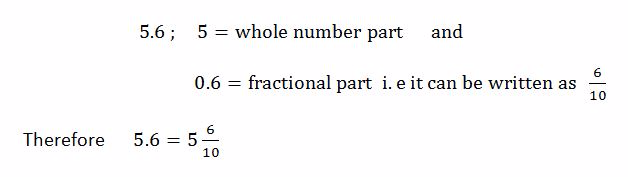Example 2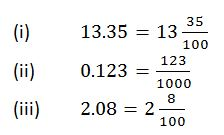Conversion of Fractions to Terminating Decimals and Vice Versa
Convert fractions to terminating decimals and vice versa
The first place after the decimal point is called tenths.

The second place after the decimal point is called hundredths e.t.c

Consider the decimal number 8.152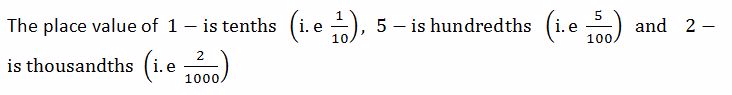NOTE

To convert a fraction into decimal, divide the numerator by denominator

To convert a decimal into fraction, write the digits after the decimal point as tenths, or hundredths or thousandths depending on the number of decimal places.

#### DECIMAL AND PERCENTAGE ~ MATHEMATICS FORM 1

Example 3

Convert the following fractions into decimals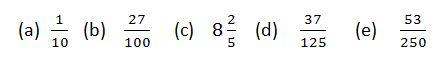Solution
Divide the numerator by denominator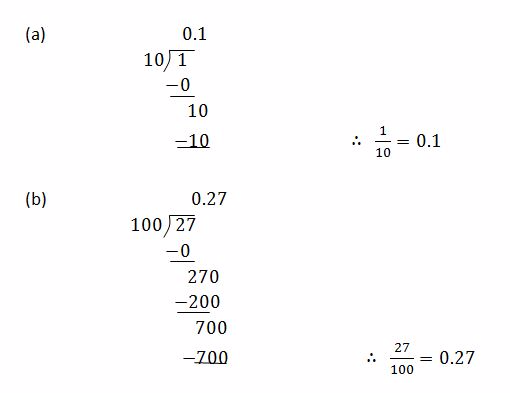#### 1 COMMENT

1.Benadetha Mwinuka

Good job